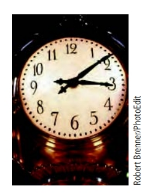Chapter 8.1, Problem 9ESMathematical Excursions (MindTap C...

4th Edition
Richard N. Aufmann + 3 others
ISBN: 9781305965584

Solutions

Chapter
SectionMathematical Excursions (MindTap C...

4th Edition
Richard N. Aufmann + 3 others
ISBN: 9781305965584
Textbook Problem

Evaluate each expression, where ⊕ and ⊖ indicate addition and subtraction, respectively, using a 12-hour clock.10 ⊖ 6

To determine

The addition 1110=9 on a 12-hour clock.

Explanation

Given information: denote the addition on 12-hour.

Calculation:

1110 denote the time 10 hours after 11 o’clock.

Therefore, 1110=9

Still sussing out bartleby?

Check out a sample textbook solution.

See a sample solution

The Solution to Your Study Problems

Bartleby provides explanations to thousands of textbook problems written by our experts, many with advanced degrees!

Get Started

Graph y=x212x a. in the standard window b. in xmin=10,xmax=30;ymin=40,ymax=40

Mathematical Applications for the Management, Life, and Social Sciences

In Exercises 5-8, find the limit. limx0(x+x)2x2x

Calculus: An Applied Approach (MindTap Course List)

Define the alpha level and the critical region for a hypothesis test.

Statistics for The Behavioral Sciences (MindTap Course List)

In Exercises 41-48, find the indicated limit given that limxaf(x)=3 and limxag(x)=4 42. limxa2f(x)

Applied Calculus for the Managerial, Life, and Social Sciences: A Brief Approach

In Exercises 3538, find the transpose of each matrix. 

Finite Mathematics for the Managerial, Life, and Social Sciences

How is the descriptive strategy different from the other four research strategies?

Research Methods for the Behavioral Sciences (MindTap Course List)

Using as an estimator, find the maximum error in estimating with the Midpoint Rule and 8 subintervals. 9

Study Guide for Stewart's Single Variable Calculus: Early Transcendentals, 8th